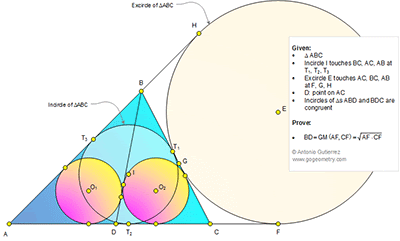## Wednesday, April 1, 2015

### Problem 1105: Triangle, Incircle, Excircle, Cevian, Tangent, Congruence, Geometric Mean

Geometry Problem. Post your solution in the comment box below.
Level: Mathematics Education, High School, Honors Geometry, College.

Click the diagram below to enlarge it.#### 1 comment:

1.This is two Sangaku with equal incircles problem . See link below for solution in details.
http://s25.postimg.org/ghxiz2xbz/two_sangaku_with_equal_incircles_problem.png

The length of cevian BD will be x= BD= sqrt(s.(s-b)) per above link.
In our case s= AF= ½ of perimeter of triangle ABC
s-b= AF-AC= CF
so BD=sqrt(AF.CF)